## How to Calculate and Solve for Mass, Velocity and Kinetic Energy | The Calculator Encyclopedia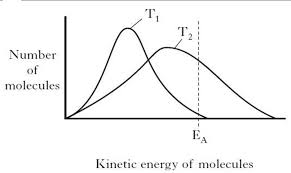The image above represents kinetic energy.

To compute for the kinetic energy, two essential parameters are needed and these parameters are mass (m) and velocity (v).

The formula for calculating the kinetic energy:

K.E. = 0.5mv²

Where;
K.E. = Kinetic Energy
m = Mass
v = Velocity

Let’s solve an example;
Find the kinetic energy when the mass is 6 and the velocity is 18.

This implies that;
m = Mass = 6
v = Velocity = 18

K.E. = 0.5mv²
K.E. = 0.5[6 x 18²]
K.E. = 0.5[6 x 324]
K.E. = 0.5
K.E. = 972

Therefore, the kinetic energy is 972 Joules (J).

Calculating the Mass when Kinetic Energy and Velocity is Given.

m = K.E / 0.5v2

Where;
m = Mass
K.E. = Kinetic Energy
v = Velocity

Let’s solve an example;
Find the mass when the kinetic energy is 320 and a velocity of 20.

This implies that;
K.E. = Kinetic Energy = 320
v = Velocity = 20

m = K.E / 0.5v2
m = 320 / 0.5 x 202
m = 320 / 0.5 x 40
m = 320 / 20
m = 16

Therefore, the mass is 16 kg.

## How to Calculate and Solve for Mass, Volume and Linear Momentum | The Calculator Encyclopedia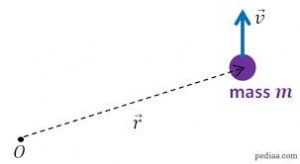The image above represents a linear momentum.

To compute for the linear momentum, two essential parameters are needed and these parameters are mass (m) and velocity (v).

The formula for calculating linear momentum:

p = mv

Where;
p = Momentum
m = Mass
v = Velocity

Let’s solve an example;
Find the linear momentum of a mass of 44 and a velocity of 38.

This implies that;
m = Mass = 44
v = Velocity = 38

p = mv
p = 44 x 38
p = 1672

Therefore, the linear momentum is 1672 Kgm/s.

Calculating the Mass when Linear Momentum and Velocity is Given.

m = p / v

Where;
m = Mass
p = Momentum
v = Velocity

Let’s solve an example;
Find the mass with a linear momentum of 320 and a velocity of 80.

This implies that;
p = Momentum = 320
v = Velocity = 80

m = p / v
m = 320 / 80
m = 4

Therefore, the mass is 4 kg.

## How to Calculate and Solve for the Quantity of Charge, Electrochemical Equivalence of a Substance and Mass of an Element in Electrolysis | Nickzom Calculator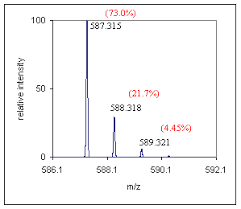The image above represents the mass of an element.

To compute for the mass of an element, two essential parameters are needed and these parameters are Electrochemical Equivalence of the Substance (Z) and quantity of charge (Q).

The formula for calculating mass of an element:

M = ZQ

Where;
M = Mass of the element
Z = Electrochemical Equivalence of the Substance
Q = Quantity of Charge

Let’s solve an example;
Find the mass of an element when the Quantity of charge is 28 and Electrochemical Equivalence of the Substance is 32.

This implies that;
Z = Electrochemical Equivalence of the Substance = 32
Q = Quantity of Charge = 28

M = ZQ
M = 32 x 28
M = 896

Therefore, the mass of an element is 896 kg.

Calculating the Electrochemical Equivalence of the Substance when the Mass of an Element and Quantity of Charge is Given.

Z = M / Q

Where;
Z = Electrochemical Equivalence of the Substance
M = Mass of the element
Q = Quantity of Charge

Let’s solve an example;
Find the Electrochemical Equivalence of the Substance when the Quantity of charge is 12 and  mass of an element  is 120.

This implies that;
M = Mass of the element = 120
Q = Quantity of Charge = 12

Z = M / Q
Z = 120 / 12
Z = 10

Therefore, the Electrochemical Equivalence of the Substance is 10.

## How to Calculate and Solve for the Current, Time and Quantity of Charge of an Electrolysis | The Calculator Encyclopedia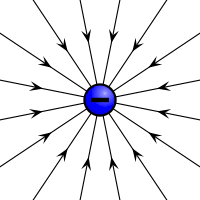The image above represents the quantity of charge.

To compute for the quantity of charge, two essential parameters are needed and these parameters are current (I) and time (T).

The formula for calculating the quantity of charge:

Q = It

Where;
Q = Quantity of charge
I = Current
T = Time

Let’s solve an example;
Find the quantity of charge with a current of 24 and time of 12.

This implies that;
I = Current = 24
T = Time = 12

Q = It
Q = 24 x 12
Q = 288

Therefore, the quantity of charge is 288 Coulombs (C).

Calculating the Current (I) using the Quantity of Charge and Time.

I = Q / t

Where;
I = Current
Q = Quantity of charge
T = Time

Let’s solve an example;
Given that the quantity of charge is 240 with a time of 14. Find the Current?

This implies that;
Q = Quantity of charge = 240
T = Time = 14

I = Q / t
I = 240 / 14
I = 17.14

Therefore, the current is 17.14 ampere.

## How to Calculate and Solve for Mass, Volume and Density | The Calculator Encyclopedia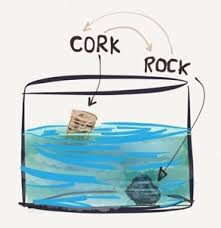The image above represents density.

To compute for the density, two essential parameters are needed and these parameters are mass (m) and volume (v).

The formula for calculating density:

Density = mass / volume

Let’s solve an example;
Given that the volume is 20 m³ with a mass of 240 kg. Find the density?

This implies that;
Volume = 20
Mass = 240

Density = mass / volume
Density = 240 / 20
Density = 12

Therefore, the density is 12 Kg/m³.

Calculating the Mass when the Density and Volume is Given.

Mass = Volume x Density

Let’s solve an example;
With a density of 90 kg/m³ and a volume of 15 m³, Find the mass?

This implies that;
Density = 90
Volume = 15

Mass = Volume x Density
Mass = 15 x 90
Mass = 1350

Therefore, the mass is 1350 kg.

## How to Calculate and Solve for Magnetic Flux Density or Field Induction | The Calculator Encyclopedia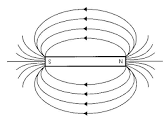The image above represents the magnetic flux density/field induction.

To compute the magnetic force of a field, four essential parameters are needed and the parameters are Magnetic Force (F), Quantity of Charge (q), Average Velocity of the Charge (v) and Angle between v and B (θ).

The formula for calculating the magnetic flux density/field induction:

B = F / qVsinθ

Where;
B = Magnetic Induction or Magnetic Flux Density
F = Magnetic Force
q = Quantity of Charge
v = Average Velocity of the charge
θ = Angle between v and B

Let’s solve an example;
Find the magnetic flux density/field induction of a field when Magnetic Force (F) is 17, Quantity of Charge (q) is 21, Average Velocity of the Charge (v) is 15 and Angle between v and B (θ) is 120°.

This implies that;
F = Magnetic Force = 17
q = Quantity of Charge = 21
v = Average Velocity of the charge = 15
θ = Angle between v and B = 120°

B = F / qVsinθ
B = 17 / (21 x 15)(sin 120°)
B = 17 / (315)(0.866)
B = 17 / (272.79)
B = 0.0623

Therefore, the magnetic flux density/field induction is 0.0623 Tesla.

## How to Calculate and Solve for the Current, Time and Quantity of Charge | Nickzom Calculator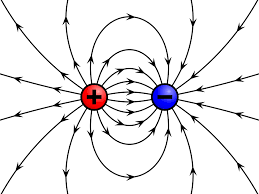The image above represents the quantity of charge.

To compute the quantity of charge, two essential parameters are needed and the parameters are current (I) and time (t).

The formula for calculating the quantity of charge;

Q = It

Where;
Q = Quantity of Charge
I = Current
t = Time

Let’s solve an example;
Find the quantity of charge when the current (I) is 24 amp with a time of 8 secs.

This implies that;
I = Current = 24 amp
t = Time = 8 secs

Q = It
Q = 24 x 8
Q = 192

Therefore, the quantity of charge is 192 coulombs (C).

Calculating the Current (I) of a charge using the Quantity of Charge (Q) and the time (t).

I = Q / t

Where;
I = Current
Q = Quantity of the charge
t = Time

Let’s solve an example;
Find the current of a charge with the quantity of the charge as 150 coulombs (C) and time as 15 secs.

This implies that;
Q = Quantity of the charge = 150 C
t = Time = 15 secs

I = Q / t
I = 150 / 15
I = 10

Therefore. the current is 10 Ampere (A).

## How to Calculate and Solve for Magnetic Force | Nickzom Calculator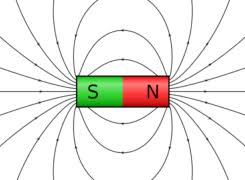The image above represents magnetic force.

To compute the magnetic force of a field, four essential parameters are needed and the parameters are Quantity of Charge (q), Average Velocity of the Charge (v), Magnetic Field Induction or Magnetic Flux Density (B) and Angle between v and B (θ).

The formula for calculating the magnetic force:

F = qVBsinθ

Where;
F = Magnetic Force
q = Quantity of Charge
v = Average Velocity of the Charge
B = Magnetic Field Induction or Magnetic Flux Density
θ = Angle between v and B

Let’s solve an example;
Find the magnetic force of a field when the Quantity of Charge (q) is 11, Average Velocity of the Charge (v) is 20, Magnetic Field Induction or Magnetic Flux Density (B) is 17 and Angle between v and B (θ) is 28°.

This implies that;
q = Quantity of Charge = 11
v = Average Velocity of the Charge = 20
B = Magnetic Field Induction or Magnetic Flux Density = 17
θ = Angle between v and B = 28°

F = qVBsinθ
F = 11 x 20 x 17 x sin28°
F = 11 x 20 x 17 x 0.469
F = 1755.82

Therefore, the magnetic force is 1755.82 Newton (N).

## How to Calculate and Solve for Escape Velocity | The Calculator Encyclopedia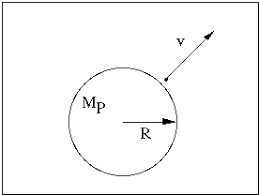The image above represents the escape velocity.

To compute the escape velocity of a field, two essential parameters are needed and the parameters are acceleration due to gravity (g) and radius (r).

The formula for calculating the escape velocity:

V = √(2gR)

Where;
V = Escape velocity
g = Acceleration due to gravity

Let’s solve an example;
Find the escape velocity of a field when the acceleration due to gravity is 12 and the radius is 24 cm.

This implies that;
g = Acceleration due to gravity = 12
r = Radius = 24 cm

V = √(2gR)
V = √(2 x 12 x 24)
V = √(576)
V = 24

Therefore, the escape velocity is 24 m/s.

## How to Calculate and Solve for Gravitational Potential | The Calculator Encyclopedia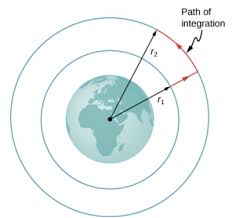The image above represents the Gravitational potential.

To compute the gravitational potential of a field, two essential parameters are needed which are mass (m) and radius (r).

The formula for calculating the gravitational potential;

V = (Gm) / r

Where;
V = Gravitational potential
m = Mass

Let’s solve an example;
Find the gravitational potential of a field when the mass is 14 cm with a radius of 9 cm.

This implies that;
m = Mass = 14 cm
r = Radius = 9 cm

V = (Gm) / r
V = (6.67 x 10-11 x 14) / 9
V = 1.0375e-10 / 9
V = 1.0375e-10

Therefore, the gravitational potential is 1.0375e-10 Volts (V).